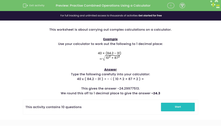# Practise Combined Operations Using a Calculator

In this worksheet, students use a calculator to carry out more complex calculations.Key stage:  KS 3

Curriculum topic:   Number

Curriculum subtopic:   Use Calculators/Technology for Accuracy

Difficulty level:#### Worksheet Overview

This worksheet is about carrying out complex calculations on a calculator.

Example

Use your calculator to work out the following to 1 decimal place:

 40 × (84.2 - 31) √ (102 + 872 )

Type the following carefully into your calculator:

40 x ( 84.2 - 31 ) ÷  √ ( 10 ^ 2 + 87 ^ 2 ) =